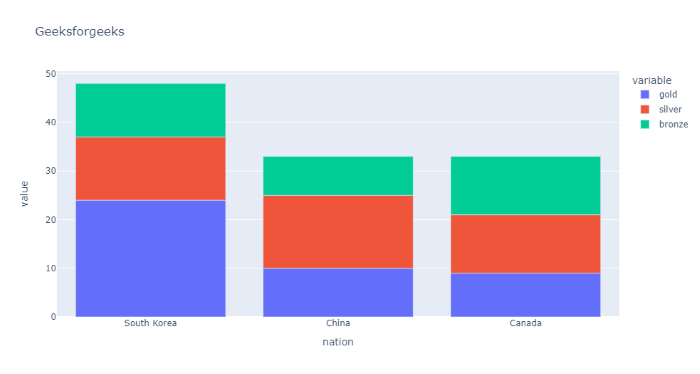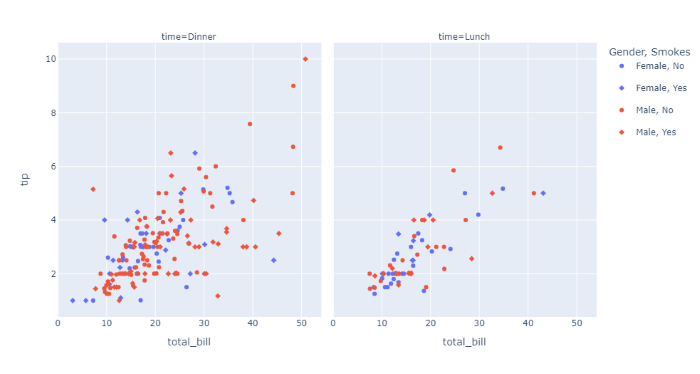Open in App
Not now

# Set the amount of vertical space between legend groups using Plotly-Python

• Last Updated : 22 Nov, 2021

In this article, we will learn how to set the amount of vertical space between legend groups.

A legend is an area describing the elements of the graph. In the plotly legend is used to Place a legend on the axes.

Example 1: In this Bar chart, We are setting the amount of vertical space (in px) between legend groups with the help of a method called fig.update_layout(legend_tracegroupgap= 2), by passing the legend_tracegroupgap parameter as 2 pixels.

## Python3

 `# importing packages ` `import` `plotly.express as px ` ` `  `# using medals_wide dataset ` `wide_df ``=` `px.data.medals_wide() ` ` `  `# plotting the bar chart ` `fig ``=` `px.bar(wide_df, x``=``"nation"``, y``=``[ ` `             ``"gold"``, ``"silver"``, ``"bronze"``], ` `             ``title``=``"Geeksforgeeks"``) ` ` `  `# spacing legend in plotly in pixels. ` `fig.update_layout(legend_tracegroupgap``=``2``) ` ` `  `# showing fig. ` `fig.show() `

Output:Example 2: In this Scatter chart, We are setting the amount of vertical space (in px) between legend groups with the help of a method called fig.update_layout(legend_tracegroupgap= 2), by passing the legend_tracegroupgap parameter as 12 pixels.

## Python3

 `# importing packages ` `import` `plotly.express as px ` ` `  `# using the gapminder dataset ` `df ``=` `px.data.tips() ` `fig ``=` `px.scatter(df, x``=``"total_bill"``, y``=``"tip"``, color``=``"sex"``, ` `                 ``symbol``=``"smoker"``, facet_col``=``"time"``, ` `                 ``labels``=``{``"sex"``: ``"Gender"``, ``"smoker"``: ``"Smokes"``}) ` ` `  `# spaceing between legend in pixel. ` `fig.update_layout(legend_tracegroupgap``=``12``) ` ` `  `# showing figure. ` `fig.show() `

Output:My Personal Notes arrow_drop_up
Related Articles